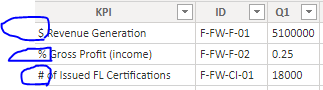cancel
Showing results for
Did you mean:New Member

## how to formatting a column in power bi have number and percentage

how to formatting a column in power bi have number and percentage in the same column

1 ACCEPTED SOLUTIONCommunity Champion

Hello @Abasalm

and use this for a little more professional formatting of the text 🙂

For other type of formatting (I integrated only % and \$) you have to expand the if statement

``````let
Source = Table.FromRows(Json.Document(Binary.Decompress(Binary.FromText("i45WUlEoSS0uUdJRMjQAA6VYnWglVZiggY6RqVJsLAA=", BinaryEncoding.Base64), Compression.Deflate)), let _t = ((type nullable text) meta [Serialized.Text = true]) in type table [KPI = _t, Q1 = _t]),
#"Changed Type" = Table.TransformColumnTypes(Source,{{"KPI", type text}, {"Q1", Percentage.Type}}),
FormatQ1 = Table.TransformRows
(
#"Changed Type",
(rec)=>
if Text.Start(rec[KPI],1)="\$" then   Record.TransformFields(rec,{"Q1",each Number.ToText(_, "n") & " \$" }) else if Text.Start(rec[KPI],1)="%" then   Record.TransformFields(rec,{"Q1", each Number.ToText(_, "P1")}) else rec
),

RecordsTobTable = Table.FromRecords(FormatQ1)
in
RecordsTobTable``````

Copy paste this code to the advanced editor in a new blank query to see how the solution works.

If this post helps or solves your problem, please mark it as solution (to help other users find useful content and to acknowledge the work of users that helped you)
Kudoes are nice too

Have fun

Jimmy

6 REPLIES 6Community Champion

Hello @Abasalm

can you make an example? WIth percentage you mean the symbol and you want to delete it and use only the number part?

If this so you can use this example

``````let
Source = Table.FromRows(Json.Document(Binary.Decompress(Binary.FromText("i45WMlRVio0FAA==", BinaryEncoding.Base64), Compression.Deflate)), let _t = ((type nullable text) meta [Serialized.Text = true]) in type table [NumberPercentage = _t]),
ChangePercentage = Table.TransformColumnTypes(Source,{{"NumberPercentage", Percentage.Type}})
in
ChangePercentage``````

if you have two values in one cell, then you have make an exact data example with the expected result

Copy paste this code to the advanced editor in a new blank query to see how the solution works.

If this post helps or solves your problem, please mark it as solution (to help other users find useful content and to acknowledge the work of users that helped you)
Kudoes are nice too

Have fun

JimmyNew Member

this an example for it

I have in one column different categories, how I do formating for itCommunity Champion

Hello @Abasalm

just to be clear... you want to format the column Q1 according to the symbol that is stated as first character in your KPI column?

JimmyNew MemberCommunity Champion

Hello @Abasalm

and use this for a little more professional formatting of the text 🙂

For other type of formatting (I integrated only % and \$) you have to expand the if statement

``````let
Source = Table.FromRows(Json.Document(Binary.Decompress(Binary.FromText("i45WUlEoSS0uUdJRMjQAA6VYnWglVZiggY6RqVJsLAA=", BinaryEncoding.Base64), Compression.Deflate)), let _t = ((type nullable text) meta [Serialized.Text = true]) in type table [KPI = _t, Q1 = _t]),
#"Changed Type" = Table.TransformColumnTypes(Source,{{"KPI", type text}, {"Q1", Percentage.Type}}),
FormatQ1 = Table.TransformRows
(
#"Changed Type",
(rec)=>
if Text.Start(rec[KPI],1)="\$" then   Record.TransformFields(rec,{"Q1",each Number.ToText(_, "n") & " \$" }) else if Text.Start(rec[KPI],1)="%" then   Record.TransformFields(rec,{"Q1", each Number.ToText(_, "P1")}) else rec
),

RecordsTobTable = Table.FromRecords(FormatQ1)
in
RecordsTobTable``````

Copy paste this code to the advanced editor in a new blank query to see how the solution works.

If this post helps or solves your problem, please mark it as solution (to help other users find useful content and to acknowledge the work of users that helped you)
Kudoes are nice too

Have fun

JimmyCommunity Champion

Hello @Abasalm

try this.It uses Table.TransformRows to check the first character of your KPI column and then it adds it as text to the Q1 column.

You could work also with a Table.AddColumn to do the same. This is only for visual, as the cell type is text.

Here the complete code

``````let
Source = Table.FromRows(Json.Document(Binary.Decompress(Binary.FromText("i45WUlEoSS0uUdJRMjQAA6VYnWglVZiggY6RqVJsLAA=", BinaryEncoding.Base64), Compression.Deflate)), let _t = ((type nullable text) meta [Serialized.Text = true]) in type table [KPI = _t, Q1 = _t]),
#"Changed Type" = Table.TransformColumnTypes(Source,{{"KPI", type text}, {"Q1", Percentage.Type}}),
FormatQ1 = Table.TransformRows
(
#"Changed Type",
(rec)=>
if Text.Start(rec[KPI],1)="\$" then   Record.TransformFields(rec,{"Q1",each Text.From(_) & " \$"}) else if Text.Start(rec[KPI],1)="%" then   Record.TransformFields(rec,{"Q1",each Text.From(Number.From(_)*100) & " %"}) else rec
),

RecordsTobTable = Table.FromRecords(FormatQ1)
in
RecordsTobTable``````

Copy paste this code to the advanced editor in a new blank query to see how the solution works.

If this post helps or solves your problem, please mark it as solution (to help other users find useful content and to acknowledge the work of users that helped you)
Kudoes are nice too

Have fun

Jimmy